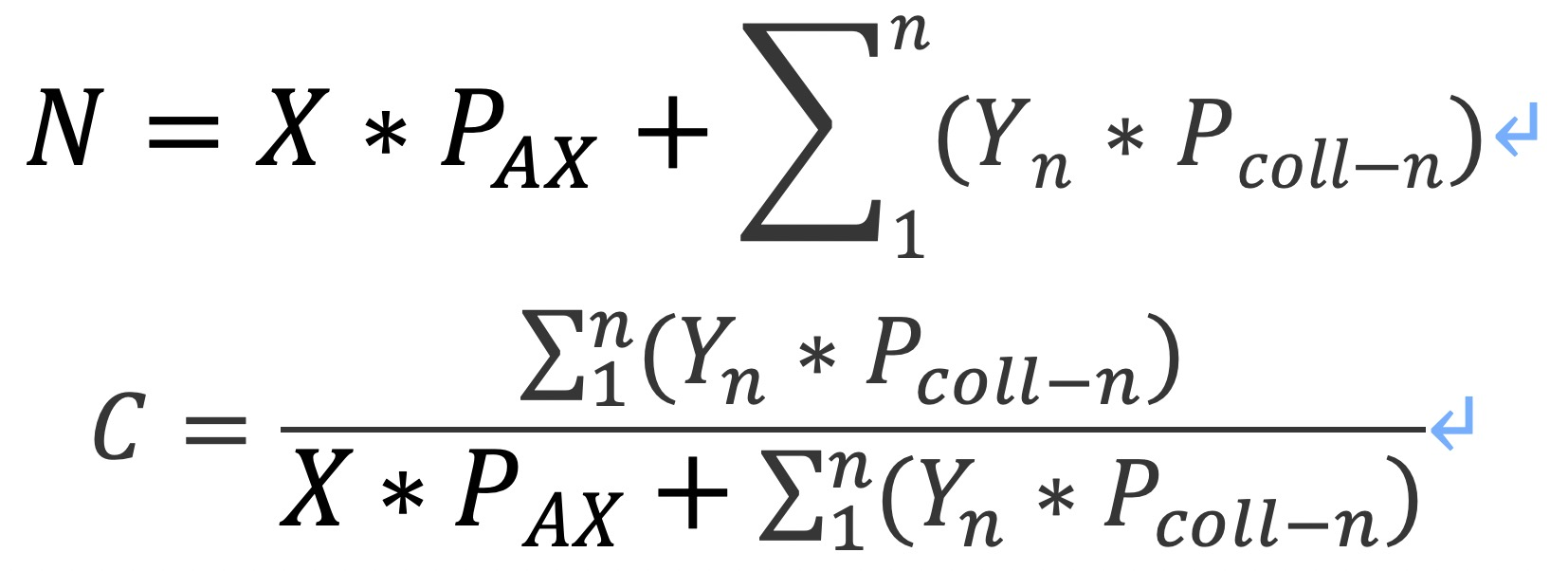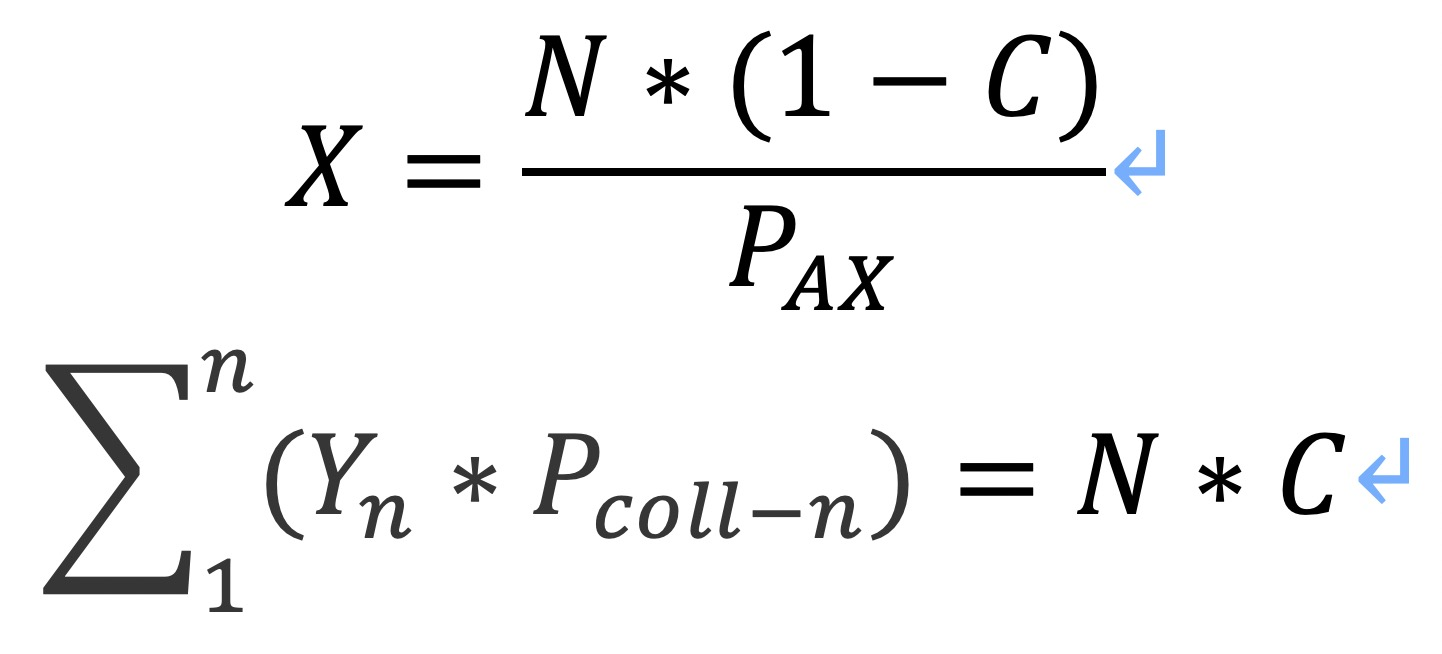### APEX: AI-Algorithmic-Stablecoin-to-Foreign-Currency Exchange Protocol(Part 2)

Generally speaking, stablecoins on APEX go through two cycles: the inflationary cycle and the deflationary cycle. In an inflationary cycle, APEX will reduce the percentage of stakes but raise the percentage of AX required to mint an algorithmic stablecoin. While in a deflationary cycle, APEX will raise the percentage of stakes, and people redeeming their AX will get more AX and fewer stakes.

The functions contained in stablecoin contracts will calculate and update the smart adjustment process on an hourly basis, and the percentage of stakes will be adjusted by 0.25% each time. Take AUSD for example. When its price is higher than \$1, the percentage of stakes will be adjusted down 0.25% for each passing hour; and when its price is higher than \$1, the stake percentage will go up by 0.25% every hour. If the price of AUSD should stay under \$1 for a long time, the percentage of stakes will gradually move close to 100%.

##### Minting

Every type of algorithmic stablecoins has its independent contract and staking pool on APEX. Stablecoins of the same type are interchangeable and have an identical stake percentage regardless of what percentage they had at the time of minting.

APEX’s functions for minting are as follows.Definition of Symbols

N is the number of target algorithmic stablecoins minted;

X is the number of AX that are burnt for minting stablecoins;

P_AX is the current price of AX;

∑_1^n〖(Y_n*P_(coll-n))〗 is the total value of the assets staked for minting;

Y_n is the number of the nth type of stakes required for minting;

P_(coll-n) is the current price of the nth type of stakes;

C is the current stake ratio.

Take AUSD for example. If we have C=97%, then to mint an AUSD requires \$0.97 worth of stakes and burns \$0.03 worth of AX.

Note that on APEX minting algorithmic stablecoins costs a minting fee, which is charged in AX at 0.2% of the total value of the minted stablecoins.

##### Redemption

The functions for redemption are as below.Definition of Symbols

N is the number of target algorithmic stablecoins redeemed;

X is the number of AX that people would receive as a result of redemption;

P_AX is the current price of AX;

Y_n is the number of the nth type of stakes in the redemption process;

P_(coll-n) is the current price of the nth type of stakes;

C is the current stake ratio;

∑_1^n〖(Y_n*P_(coll-n))〗 is the total value of the stakes users would receive as a result of redemption.

Note that on APEX redeeming algorithmic stablecoins costs a fee, which is charged in AX at 0.45% of the total value redeemed.